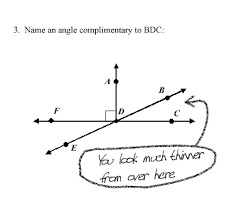Midsegment Theorem
4 years ago
kgiraldi
Save
Edit
Host a game
Live GameLive
Homework
Solo Practice
Practice10 QuestionsShow answers
• Question 1
30 secondsQ. Is DE parallel to BC?
Yes
No
• Question 2
30 secondsQ. Is DE 1/2 the length of BC?
Yes
No
• Question 3
30 secondsQ. Find the length of AB
6
11
17
68
• Question 4
120 secondsQ. Find the length of AB
6
11
17
68
• Question 5
60 secondsQ. What is the length of AB?
4
8
16
24
• Question 6
120 secondsQ. What is the value of x?
10
No Solution
5
2
• Question 7
120 secondsQ. What is the length of the Midsegment?
6
9
12
18
• Question 8
120 secondsQ. What is the value of y?
3
-3
No Solution
5
• Question 9
120 secondsQ.

What is the length of right side of the triangle?

12

24

10

20

• Question 10
60 secondsQ. What is the measure of angle a?